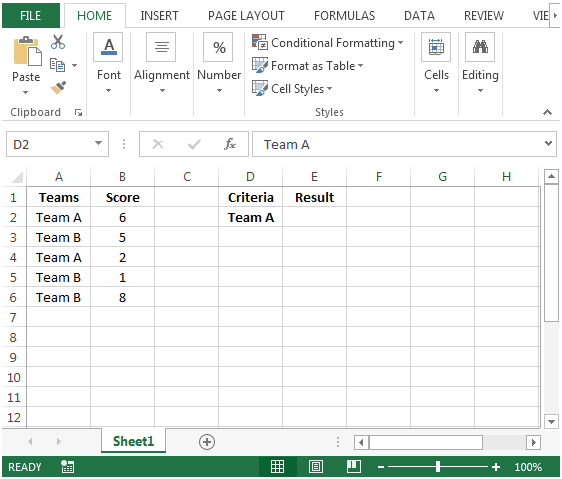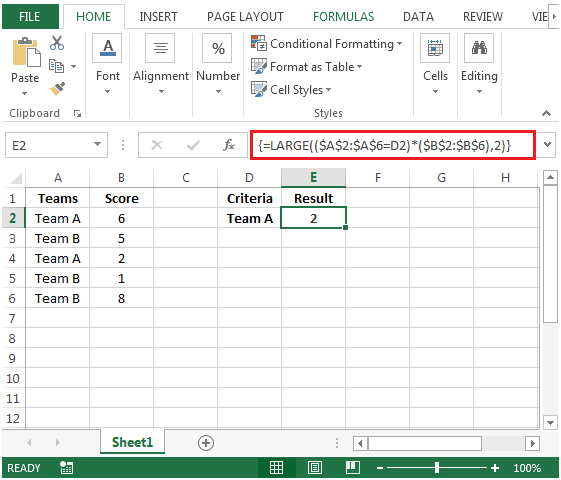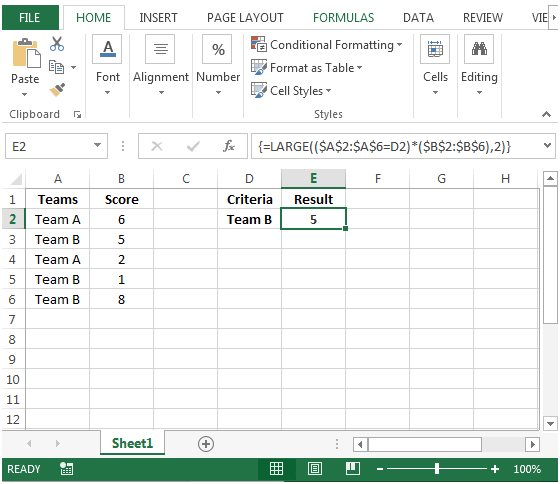# Finding the Score that Was the Nth Highest for a Specified Team in Microsoft Excel 2010

To find the nth highest score for a specified team, we can use the LARGE function to retrieve the result.

LARGE: Returns the k-th largest value in a data set. For example: the second largest number from a list of 10 items.

Syntax: =LARGE(array,k)

array: is an array or range of cells in a list of data for which you need to find the k-th largest value.

k: is the kth position from largest value to return in the array or range of cells.

Let us take an example:

Column A contains couple of teams & column B contains their respective scores.We need a formula to derive the second highest score based on the selected team in cell D2.• In cell E2, the formula would be
• =LARGE((\$A\$2:\$A\$6=D2)*(\$B\$2:\$B\$6),2)
• This is an array formula that requires formula to be enclosed with curly brackets by using CTRL + SHIFT + ENTER.
• {=LARGE((\$A\$2:\$A\$6=D2)*(\$B\$2:\$B\$6),2)}
• Here 2 is the 2nd largest number for Team A.  Hence, the result is 2.• If we change the criteria in cell D2 from team A to team B then the formula will automatically update the result accordingly.
• For Team B, there are 3 scores, 5, 1, 8 and 5 is the 2nd largest number, and hence the output is 5.Terms and Conditions of use

The applications/code on this site are distributed as is and without warranties or liability. In no event shall the owner of the copyrights, or the authors of the applications/code be liable for any loss of profit, any problems or any damage resulting from the use or evaluation of the applications/code.